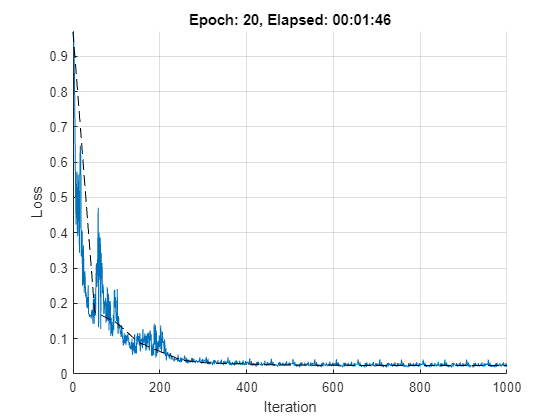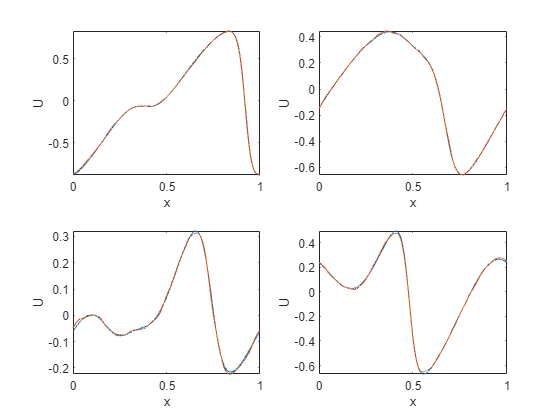## fourier-neural-operator

Version 1.0.0 (48.5 KB) by
The Fourier Neural Operator is a neural operator with an integral kernel parameterized in Fourier space.

Updated 12 Jan 2023

From GitHub

# Fourier Neural OperatorThe Fourier Neural Operator (FNO)  is a neural operator with an integral kernel parameterized in Fourier space. This allows for an expressive and efficient architecture. Applications of the FNO include weather forecasting and, more generically, finding efficient solutions to the Navier-Stokes equations which govern fluid flow.

## Setup

Add fno directory to the path.

addpath(genpath('fno'));

Requires:

## References

 Zongyi Li, Nikola Kovachki, Kamyar Azizzadenesheli, Burigede Liu, Kaushik Bhattacharya, Andrew Stuart, and Anima Anandkumar. Fourier Neural Operator for Parametric Partial Differential Equations. In International Conference on Learning Representations (ICLR), 2021a. (https://openreview.net/forum?id=c8P9NQVtmnO)

# Example: Fourier Neural Operator for 1d Burgers' Equation

In this example we apply the Fourier Neural Operator to learn the one-dimensional Burgers' equation with the following definition:

## Data preparation

We use the burgers_data_R10.mat, which contains initial velocitiesand solutionsof the Burgers' equation. We then use these as training inputs and targets respectively. The network inputs include the spatial domainat the desired discretization. In this example we choose a grid size of.

% Setup.

end
if ~exist(dataFile, 'file')
location = 'https://ssd.mathworks.com/supportfiles/nnet/data/burgers1d/burgers_data_R10.mat';
websave(dataFile, location);
end
x = data.a;
t = data.u;

% Specify the number of observations in training and test data, respectively.
numTrain = 1e3;
numTest = 1e2;

% Specify grid size and downsampling factor.
h = 2^10;
n = size(x,2);
ns = floor(n./h);

% Downsample the data for training.
xTrain = x(1:numTrain, 1:ns:n);
tTrain = t(1:numTrain, 1:ns:n);
xTest = x(end-numTest+1:end, 1:ns:n);
tTest = t(end-numTest+1:end, 1:ns:n);

% Define the grid over the spatial domain x.
xmax = 1;
xgrid = linspace(0, xmax, h);

% Combine initial velocities and spatial grid to create network
% predictors.
xTrain = cat(3, xTrain, repmat(xgrid, [numTrain 1]));
xTest = cat(3, xTest, repmat(xgrid, [numTest 1]));


## Define network architecture

Here we create a dlnetwork for the Burgers' equation problem. The network accepts inputs of dimension [h 2 miniBatchSize], and returns outputs of dimension [h 1 miniBatchSize]. The network consists os multiple blocks which combine spectral convolution with regular, linear convolution. The convolution in Fourier space filters out higher order oscillations in the solution, while the linear convolution learns local correlations.

numModes = 16;
width = 64;

lg = layerGraph([ ...
convolution1dLayer(1, width, Name='fc0')

spectralConvolution1dLayer(width, numModes, Name='specConv1')
reluLayer(Name='relu1')

spectralConvolution1dLayer(width, numModes, Name='specConv2')
reluLayer(Name='relu2')

spectralConvolution1dLayer(width, numModes, Name='specConv3')
reluLayer(Name='relu3')

spectralConvolution1dLayer(width, numModes, Name='specConv4')

convolution1dLayer(1, 128, Name='fc5')
reluLayer(Name='relu5')
convolution1dLayer(1, 1, Name='fc6')
]);

lg = addLayers(lg, convolution1dLayer(1, width, Name='fc1'));
lg = connectLayers(lg, 'fc0', 'fc1');

lg = addLayers(lg, convolution1dLayer(1, width, Name='fc2'));
lg = connectLayers(lg, 'relu1', 'fc2');

lg = addLayers(lg, convolution1dLayer(1, width, Name='fc3'));
lg = connectLayers(lg, 'relu2', 'fc3');

lg = addLayers(lg, convolution1dLayer(1, width, Name='fc4'));
lg = connectLayers(lg, 'relu3', 'fc4');

numInputChannels = 2;
XInit = dlarray(ones([h numInputChannels 1]), 'SCB');
net = dlnetwork(lg, XInit);

analyzeNetwork(net)


## Training options

The network is trained using the standard SGDM algorithm, where the learn rate is decreased every stepSize iterations.

executionEnvironment = "gpu";

batchSize = 20;
learnRate = 1e-3;
momentum = 0.9;

numEpochs = 20;
stepSize = 100;
gamma = 0.5;
expNum = 1;
checkpoint = false;
expDir = sprintf( 'checkpoints/run%g', expNum );
if ~isfolder( expDir ) && checkpoint
mkdir(expDir)
end

vel = [];
totalIter = 0;

numTrain = size(xTrain,1);
numIterPerEpoch = floor(numTrain./batchSize);


## Training loop

Train the network.

if executionEnvironment == "gpu" && canUseGPU
xTrain = gpuArray(xTrain);
xTest = gpuArray(xTest);
end

start = tic;
figure;
clf
lineLossTrain = animatedline('Color', [0 0.4470 0.7410]);
lineLossTest = animatedline('Color', 'k', 'LineStyle', '--');
ylim([0 inf])
xlabel("Iteration")
ylabel("Loss")
grid on

% Compute initial validation loss.
y = net.predict( dlarray(xTest, 'BSC') );
yTest = extractdata(permute(stripdims(y), [3 1 2]));
relLossTest = relativeL2Loss(yTest , tTest);

% Main loop.
lossfun = dlaccelerate(@modelLoss);
for epoch = 1:numEpochs
% Shuffle the data.
dataIdx = randperm(numTrain);

for iter = 1:numIterPerEpoch
% Get mini-batch data.
batchIdx = (1:batchSize) + (iter-1)*batchSize;
idx = dataIdx(batchIdx);
X = dlarray( xTrain(batchIdx, :, :), 'BSC' );
T = tTrain(batchIdx, :);

[loss, dnet] = dlfeval(lossfun, X, T, net);

% Update model parameters using SGDM update rule.
[net, vel] = sgdmupdate(net, dnet, vel, learnRate, momentum);

% Plot training progress.
totalIter = totalIter + 1;
D = duration(0,0,toc(start),'Format','hh:mm:ss');
title("Epoch: " + epoch + ", Elapsed: " + string(D))
drawnow

% Learn rate scheduling.
if mod(totalIter, stepSize) == 0
learnRate = gamma.*learnRate;
end
end
% Compute validation loss and MSE.
y = net.predict( dlarray(xTest, 'BSC') );
yTest = extractdata(permute(stripdims(y), [3 1 2]));
relLossTest = relativeL2Loss( yTest , tTest );
mseTest = mean( (yTest(:) - tTest(:)).^2 );

% Display progress.
D = duration(0,0,toc(start),'Format','hh:mm:ss');
numTest = size(xTest, 1);
fprintf('Epoch = %g, train loss = %g, val loss = %g, val mse = %g, total time = %s. \n', ...
epoch, extractdata(loss)/batchSize, relLossTest/numTest, mseTest/numTest, string(D));

% Checkpoints.
if checkpoint
filename = sprintf('checkpoints/run%g/epoch%g.mat', expNum, epoch);
save(filename, 'net', 'epoch', 'vel', 'totalIter', 'relLossTest', 'mseTest', 'learnRate');
end
end

Epoch = 1, train loss = 0.226405, val loss = 0.175389, val mse = 7.73286e-05, total time = 00:00:13.
Epoch = 2, train loss = 0.153691, val loss = 0.145805, val mse = 5.99213e-05, total time = 00:00:22.
Epoch = 3, train loss = 0.0923258, val loss = 0.0904608, val mse = 2.49174e-05, total time = 00:00:27.
Epoch = 4, train loss = 0.102122, val loss = 0.0639219, val mse = 1.43723e-05, total time = 00:00:32.
Epoch = 5, train loss = 0.0346076, val loss = 0.0393621, val mse = 9.33419e-06, total time = 00:00:36.
Epoch = 6, train loss = 0.0361029, val loss = 0.032303, val mse = 7.10724e-06, total time = 00:00:45.
Epoch = 7, train loss = 0.0270364, val loss = 0.0296161, val mse = 6.43696e-06, total time = 00:00:50.
Epoch = 8, train loss = 0.0263171, val loss = 0.0283881, val mse = 5.92292e-06, total time = 00:00:54.
Epoch = 9, train loss = 0.0248211, val loss = 0.0261364, val mse = 5.54218e-06, total time = 00:00:58.
Epoch = 10, train loss = 0.0243392, val loss = 0.0253596, val mse = 5.32946e-06, total time = 00:01:03.
Epoch = 11, train loss = 0.0236119, val loss = 0.0250861, val mse = 5.22886e-06, total time = 00:01:07.
Epoch = 12, train loss = 0.023318, val loss = 0.024752, val mse = 5.12552e-06, total time = 00:01:11.
Epoch = 13, train loss = 0.0230901, val loss = 0.0243369, val mse = 5.04185e-06, total time = 00:01:16.
Epoch = 14, train loss = 0.0229644, val loss = 0.0241713, val mse = 4.99882e-06, total time = 00:01:20.
Epoch = 15, train loss = 0.0228391, val loss = 0.0240904, val mse = 4.99516e-06, total time = 00:01:25.
Epoch = 16, train loss = 0.022768, val loss = 0.0240143, val mse = 4.97173e-06, total time = 00:01:29.
Epoch = 17, train loss = 0.0228152, val loss = 0.023916, val mse = 4.95474e-06, total time = 00:01:33.
Epoch = 18, train loss = 0.022787, val loss = 0.0238792, val mse = 4.94643e-06, total time = 00:01:38.
Epoch = 19, train loss = 0.0227602, val loss = 0.023865, val mse = 4.93665e-06, total time = 00:01:42.
Epoch = 20, train loss = 0.0227464, val loss = 0.0238451, val mse = 4.93358e-06, total time = 00:01:46.## Test on unseen, higher resolution data

Here we take the trained network and test on unseen data with a higher spatial resolution than the training data. This is an example of zero-shot super-resolution.

gridHighRes = linspace(0, xmax, n);

idxToPlot = numTrain+(1:4);
figure;
for p = 1:4
xn = dlarray(cat(1, x(idxToPlot(p),:), gridHighRes),'CSB');
yn = predict(net, xn);

subplot(2, 2, p)
plot(gridHighRes, t(idxToPlot(p),:)), hold on, plot(gridHighRes, extractdata(yn))
axis tight
xlabel('x')
ylabel('U')
end### Helper functions

function [loss, grad] = modelLoss(x, t, net)
y = net.forward(x);
y = permute(stripdims(y), [3 1 2]);
y = stripdims(y);

loss = relativeL2Loss(y, t);

end

function loss = relativeL2Loss(y, t)
diffNorms = normFcn( (y - t) );
tNorms = normFcn( t );

loss = sum(diffNorms./tNorms, 1);
end

function n = normFcn(x)
n = sqrt( sum(x.^2, 2) );
end


### Cite As

Conor Daly (2023). fourier-neural-operator (https://github.com/matlab-deep-learning/fourier-neural-operator), GitHub. Retrieved .

##### MATLAB Release Compatibility
Created with R2022b
Compatible with any release
##### Platform Compatibility
Windows macOS Linux

### Community Treasure Hunt

Find the treasures in MATLAB Central and discover how the community can help you!

Start Hunting!